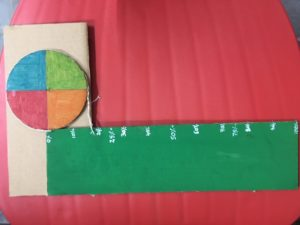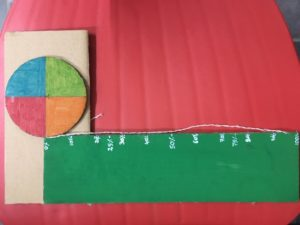# TLM for Percentage

In that we have made a rectangle from the circumference of a circle with the radius 5cm ,total height of the rectangle is 31.4cm as same as the circumference of the circle, from that we have assumed it as the 100% so that we have combined the circle at the top rectangle and it is been measured with the thread from the top of rectangle so that students can imagine the percentage and we have used to calculate the various percentage.